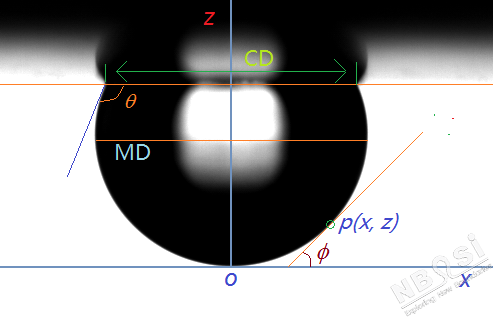# 光学俯视法接触角测量以及原理(Determination of Contact Angle by Top-Viewing a Sessile Drop)

$$\Delta p = \gamma \left( \frac{1}{R_1} + \frac{1}{R_2} \right) \tag{1} \label{1}$$

$$2 + \beta \left( \frac{z}{b} \right) = \frac{1}{R/b} + \frac{\sin\phi}{x/b} \tag{2} \label{2}$$

\begin{align} \beta &= \frac{b^2\cdot\Delta\rho\cdot g}{\gamma} = \frac{b^2}{\alpha^2} \\ \alpha &= \sqrt{\frac{\gamma}{\Delta\rho\cdot g}} \end{align} \tag{3} \label{3}

$$\Delta\rho$$ 为液滴相与周围相之间的密度差；$$g$$ 为重力加速度；$$\gamma$$ 为表面/界面张力；$$\alpha$$ 为体系的毛细管常数（capillary constant）。图1：座滴示意图

• 液滴的体积 $$v$$：它是已知的输入值；

• 液滴的接触直径CD或最大直径MD：这个数据可以通过测量得到。

Laplace-Young模型由于考虑了重力的影响，所以适合任何种类的液体（也即具有不同毛细管常数 $$\alpha$$ 的液体）和液滴体积 $$v$$ （尺寸）。图2：水滴（6μl）在磨砂有机玻璃表面的接触角测量：约133°

OSA™ Optical Surface Analyzer - SKX系列

1. S. Bashforth and J. C. Adams, An Attempt to Test the Theory of Capillary Action, Cambridge University Press and Deighton, Bell & Co., London, 1892.

2. F.K. Skinner, Y. Rotenberg and A.W. Neumann, J. Colloid Interface Sci., 130, 25 (1989).

3. E. Moy, P. Cheng, Z. Policova, S. Treppo, D. Kwok, D.P. Mack, P.M. Sherman, A.W. Neumann，Colloids and Surfaces, 58, 215 (1991).